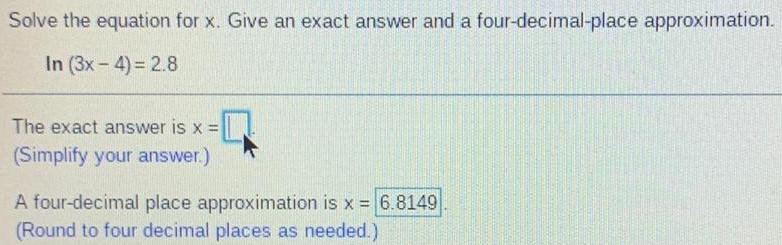Question:

# Solve the equation for x. Give an exact answer and a

Last updated: 7/8/2022Solve the equation for x. Give an exact answer and a four-decimal-place approximation. In (3x-4)= 2.8 The exact answer is x = (Simplify your answer.) A four-decimal place approximation is x = 6.8149 (Round to four decimal places as needed.)